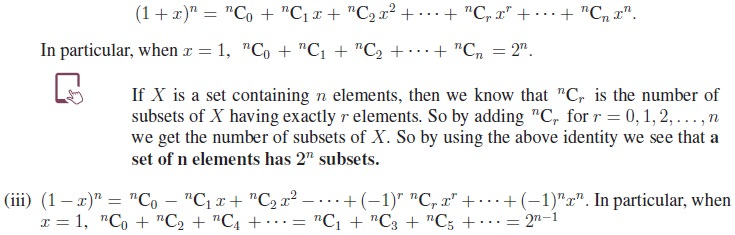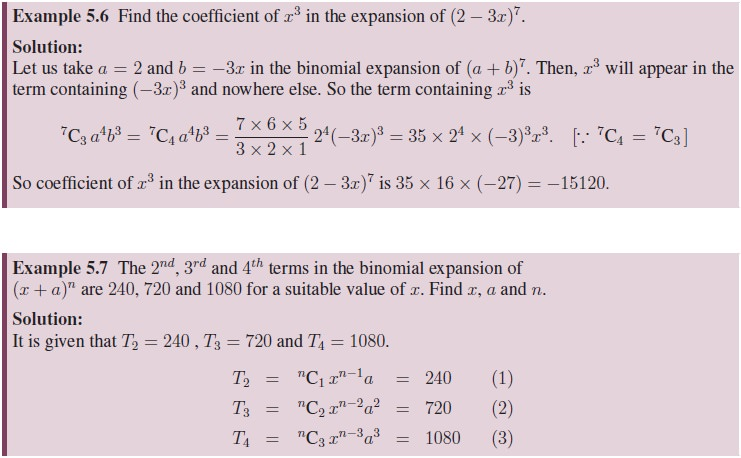Home | | Maths 11th std | Particular cases of Binomial Theorem

# Particular cases of Binomial Theorem

Mathematics : Binomial Theorem, Sequences and Series

Particular cases of Binomial Theorem

(i) Replacing b by (−b), in the binomial expansion of (a + b)n, n N, we getObserve that the sign ‘+’ and ‘−’ appear alternately in the binomial expansion of (a − b)n.

(ii) Replacing a by 1 and b by x, in the binomial expansion of (a + b)n, we getTags : Formula, Solved Example Problems, Exercise | Mathematics , 11th Mathematics : UNIT 5 : Binomial Theorem, Sequences and Series
Study Material, Lecturing Notes, Assignment, Reference, Wiki description explanation, brief detail
11th Mathematics : UNIT 5 : Binomial Theorem, Sequences and Series : Particular cases of Binomial Theorem | Formula, Solved Example Problems, Exercise | Mathematics